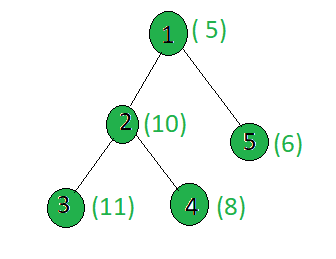# Count the nodes in the given tree whose weight is a power of two

• Last Updated : 11 Jun, 2021

Given a tree, and the weights of all the nodes, the task is to count the number of nodes whose weight is a power of 2.
Examples:

Input:Output:
Only the weight of the node 4 is a power of 2.

Approach: Perform dfs on the tree and for every node, check if its weight is a power of 2 or not, if yes then increment the count.
Below is the implementation of the above approach:

## C++

 `// C++ implementation of the approach` `#include ` `using` `namespace` `std;`   `int` `ans = 0;`   `vector<``int``> graph;` `vector<``int``> weight(100);`   `// Function to perform dfs` `void` `dfs(``int` `node, ``int` `parent)` `{` `    ``// If weight of the current node` `    ``// is a power of 2` `    ``int` `x = weight[node];` `    ``if` `(x && (!(x & (x - 1))))` `        ``ans += 1;`   `    ``for` `(``int` `to : graph[node]) {` `        ``if` `(to == parent)` `            ``continue``;` `        ``dfs(to, node);` `    ``}` `}`   `// Driver code` `int` `main()` `{`   `    ``// Weights of the node` `    ``weight = 5;` `    ``weight = 10;` `    ``weight = 11;` `    ``weight = 8;` `    ``weight = 6;`   `    ``// Edges of the tree` `    ``graph.push_back(2);` `    ``graph.push_back(3);` `    ``graph.push_back(4);` `    ``graph.push_back(5);`   `    ``dfs(1, 1);`   `    ``cout << ans;`   `    ``return` `0;` `}`

## Java

 `// Java implementation of the approach ` `import` `java.util.*;`   `class` `GFG ` `{`   `    ``static` `int` `ans = ``0``;`   `    ``@SuppressWarnings``(``"unchecked"``)` `    ``static` `Vector[] graph = ``new` `Vector[``100``];` `    ``static` `int``[] weight = ``new` `int``[``100``];`   `    ``// Function to perform dfs` `    ``static` `void` `dfs(``int` `node, ``int` `parent)` `    ``{` `        ``// If weight of the current node` `        ``// is a power of 2` `        ``int` `x = weight[node];` `        ``if` `(x != ``0` `&& (x & (x - ``1``)) == ``0``)` `            ``ans += ``1``;`   `        ``for` `(``int` `to : graph[node])` `        ``{` `            ``if` `(to == parent)` `                ``continue``;` `            ``dfs(to, node);` `        ``}` `    ``}`   `    ``// Driver Code` `    ``public` `static` `void` `main(String[] args)` `    ``{` `        ``for` `(``int` `i = ``0``; i < ``100``; i++)` `            ``graph[i] = ``new` `Vector<>();`   `        ``// Weights of the node` `        ``weight[``1``] = ``5``;` `        ``weight[``2``] = ``10``;` `        ``weight[``3``] = ``11``;` `        ``weight[``4``] = ``8``;` `        ``weight[``5``] = ``6``;`   `        ``// Edges of the tree` `        ``graph[``1``].add(``2``);` `        ``graph[``2``].add(``3``);` `        ``graph[``2``].add(``4``);` `        ``graph[``1``].add(``5``);`   `        ``dfs(``1``, ``1``);`   `        ``System.out.println(ans);` `    ``}` `}`   `// This code is contributed by` `// sanjeev2552`

## C#

 `// C# implementation of the approach ` `using` `System; ` `using` `System.Collections.Generic; `   `class` `GFG ` `{ ` `    `  `static` `int` `ans = 0; ` `static` `List> graph = ``new` `List>(); ` `static` `List<``int``> weight = ``new` `List<``int``>(); `   `// Function to perform dfs ` `static` `void` `dfs(``int` `node, ``int` `parent) ` `{ `   `    ``// If weight of the current node ` `    ``// is a power of 2 ` `    ``int` `x = weight[node]; ` `    ``bool` `result = Convert.ToBoolean((x & (x - 1)));` `    ``bool` `result1 = Convert.ToBoolean(x);` `    ``if` `(result1 && (!result)) ` `        ``ans += 1; `   `    ``for` `(``int` `i = 0; i < graph[node].Count; i++) ` `    ``{ ` `        ``if` `(graph[node][i] == parent) ` `            ``continue``; ` `        ``dfs(graph[node][i], node); ` `    ``} ` `} `   `// Driver code ` `public` `static` `void` `Main(String []args) ` `{ ` `    ``// Weights of the node ` `    ``weight.Add(0); ` `    ``weight.Add(5); ` `    ``weight.Add(10);; ` `    ``weight.Add(11);; ` `    ``weight.Add(8); ` `    ``weight.Add(6); ` `    `  `    ``for``(``int` `i = 0; i < 100; i++) ` `    ``graph.Add(``new` `List<``int``>()); `   `    ``// Edges of the tree ` `    ``graph.Add(2); ` `    ``graph.Add(3); ` `    ``graph.Add(4); ` `    ``graph.Add(5); `   `    ``dfs(1, 1); `   `    ``Console.WriteLine(ans); ` `} ` `} `   `// This code is contributed by shubhamsingh10`

## Python3

 `# Python3 implementation of the approach` `ans ``=` `0`   `graph ``=` `[[] ``for` `i ``in` `range``(``100``)] ` `weight ``=` `[``0``]``*``100`   `# Function to perform dfs ` `def` `dfs(node, parent):` `    ``global` `mini, graph, weight, ans ` `    `  `    ``# If weight of the current node ` `    ``# is a power of 2 ` `    ``x ``=` `weight[node]` `    ``if` `(x ``and` `(``not` `(x & (x ``-` `1``)))):` `        ``ans ``+``=` `1` `    ``for` `to ``in` `graph[node]: ` `        ``if` `(to ``=``=` `parent): ` `            ``continue` `        ``dfs(to, node) ` `        `  `        ``# Calculating the weighted ` `        ``# sum of the subtree ` `        ``weight[node] ``+``=` `weight[to] ` `    `  `# Driver code`   `# Weights of the node` `weight[``1``] ``=` `5` `weight[``2``] ``=` `10` `weight[``3``] ``=` `11` `weight[``4``] ``=` `8` `weight[``5``] ``=` `6`   `# Edges of the tree` `graph[``1``].append(``2``)` `graph[``2``].append(``3``)` `graph[``2``].append(``4``)` `graph[``1``].append(``5``)`   `dfs(``1``, ``1``)` `print``(ans)`   `# This code is contributed by SHUBHAMSINGH10`

## Javascript

 ``

Output:

`1`

Complexity Analysis:

• Time Complexity: O(N).
In DFS, every node of the tree is processed once and hence the complexity due to the DFS is O(N) for N nodes in the tree. Therefore, the time complexity is O(N).
• Auxiliary Space: O(1).
Any extra space is not required, so the space complexity is constant.

My Personal Notes arrow_drop_up
Recommended Articles
Page :